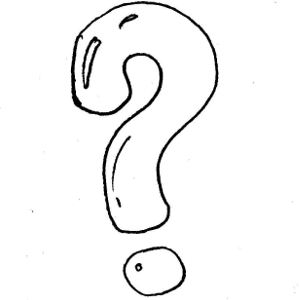# Next Number in Series

192.2K Views

Find the next number in the series below

21 32 54 87 131 ?186

21 + 11 = 32
32 + 22 = 54
54 + 33 = 87
87 + 44 = 131
131 + 55 = 186

186

The difference between the numbers is a multiplication of 11. Starts with 11 and than 22,33,44 so the next in line is 55. therefor   131+55=186